Courses
Courses for Kids
Free study material
Offline Centres
More

# NCERT Solutions for Class 10 Science Chapter 10 - Light Reflection And RefractionLast updated date: 29th Nov 2023
Total views: 473.1k
Views today: 13.72k

## NCERT Solutions for Class 10 Science Chapter 10 - Light Reflection and Refraction

NCERT Solutions for Class 10 Science Chapter 10 Light Reflection and Refraction are extremely significant towards securing a decent score in the Class 10 Science board exam. These solutions will help students during their exam preparations and in building strong foundations for the subject. NCERT Solutions for Class 10 Chapter 10 Science have been created and reviewed by some of the most experienced and knowledgeable faculties, so students need not worry about the credibility of the solutions. The Ch 10 Science Class 10 NCERT Solutions aid students in getting a hold of certain topics that have a high possibility of coming in the exam. Download NCERT Solutions for Class 10 Maths from Vedantu, which are curated by master teachers.

### Important Topics Covered in NCERT Solutions Class 10 Science Chapter 10

The important topics that are covered in this chapter are as follows.

• Reflection of Light

• Spherical Mirrors

• Image Formation by Spherical Mirrors

• Representation of Images Formed by Spherical Mirrors Using Ray Diagram

• Mirror Formula and Magnification

• Refraction of Light

• Power of Lens

Watch videos on
NCERT Solutions for Class 10 Science Chapter 10 - Light Reflection And Refraction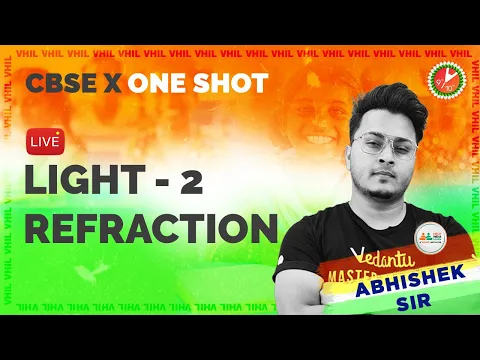Light Class 10 in One Shot Part -2 | Refraction CBSE Class 10 2023-24| Abhishek Sir @VedantuClass910
Vedantu 9&10
SubscribeShare
11.1K likes
217.5K Views
2 years ago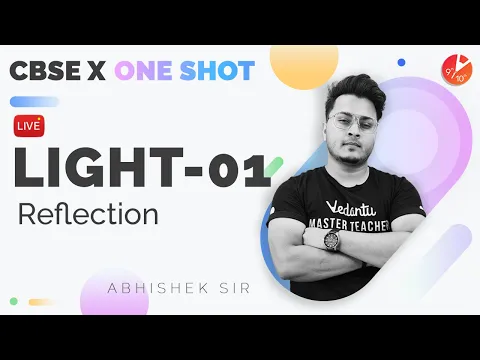Light Class 10 in One Shot | Reflection Part -1 | CBSE Class 10 | Abhishek Sir @VedantuClass910 ​
Vedantu 9&10
27.2K likes
618.5K Views
2 years ago
Play Quiz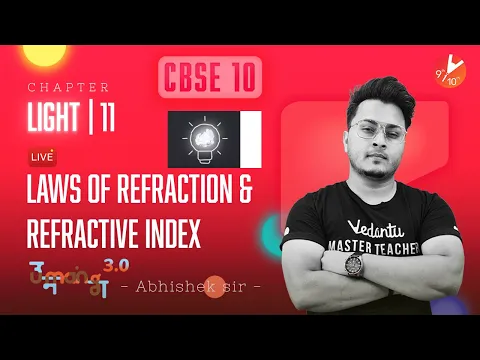Laws of Refraction and Refractive Index | Light L-11 |CBSE Class 10 Abhishek Sir @VedantuClass910
Vedantu 9&10
13.1K likes
291.2K Views
2 years ago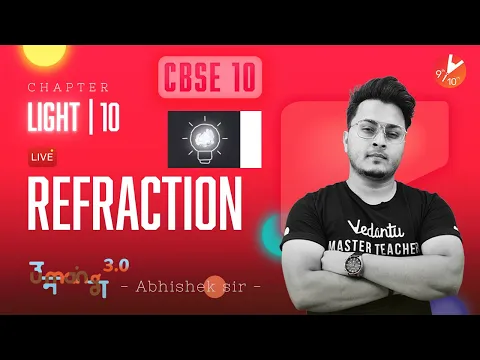Refraction | Light L-10 | CBSE Class 10 Abhishek Sir @VedantuClass910
Vedantu 9&10
14.2K likes
297K Views
2 years ago
Play Quiz
See More## Access NCERT Solutions for Class 10 Science Chapter 10 – Light Reflection and Refraction

1. What is the principal focus of a concave mirror?

Ans: The light rays parallel to the principal axis converge at a point on the principal axis after reflecting from the concave mirror.

The point of convergence on the principal axis of a concave mirror is called the principal focus of a concave mirror.

2. What is the focal length of a spherical mirror whose radius of curvature is $20cm$?

Ans: It is given that,

Radius of curvature of a spherical mirror, $R=20cm$

It is known that,

Radius of curvature is twice the focal length.

$R=2f$

$\Rightarrow f=R/2$

$\Rightarrow f=\frac{20}{2}$

$\Rightarrow f=10cm$

Therefore, the focal length of a spherical mirror with the radius of curvature equal to $20cm$ is $f=10cm$.

3. Which mirror gives an erect and enlarged image of an object?

Ans: If the object is placed between pole and the principal focus of a concave mirror the image formed is virtual, erect and enlarged.

Erect and enlarged images are not possible in case of convex or plane mirrors.

4. Why is convex mirror preferred as a rear-view mirror in vehicles?

Ans: If the objects are placed in front of a convex mirror, then the image formed is an erect and diminished image.

In vehicles we need erect images and we need to see as many areas as possible behind the vehicles.

So, a convex mirror is preferred as a rear-view mirror in vehicles.

5. What is the focal length of a convex mirror whose radius of curvature is $32cm$?

Ans: It is given that,

Radius of curvature of a convex mirror, $R=32cm$

It is known that,

Radius of curvature is twice the focal length.

$R=2f$

$\Rightarrow f=R/2$

$\Rightarrow f=\frac{32}{2}$

$\Rightarrow f=16cm$

Therefore, the focal length of a convex mirror with the radius of curvature equal to $32cm$ is $f=16cm$.

6. Find the location of image for a concave mirror that produces three times magnified (enlarged) real image of the object placed at $10cm$ in front of it.

Ans: It is given that,

Distance of object in front of mirror, $u=-10cm$ (negative sign due to the location of the object in front of the mirror)

Distance of image from mirror, $v=?$

It is known that,

Magnification of a spherical mirror,$m=\frac{{{h}_{i}}}{{{h}_{o}}}=-\frac{v}{u}$

where,

${{h}_{i}}$ is the height of the image

${{h}_{o}}$ is the height of the object

Let, ${{h}_{o}}=h$

It is given that three times the enlarged real image of the object is produced.

So, ${{h}_{i}}=-3h$ ($-$ due to real image formation)

$\Rightarrow m=-\frac{3h}{h}=-\frac{v}{u}$

$\Rightarrow 3=\frac{v}{-10}$

$\Rightarrow v=-30cm$(negative sign due to the formation of inverted image)

Therefore, the location of image from the mirror is at a distance of $30cm$ and the nature of the image is inverted.

7. When a light ray travelling in the air enters obliquely into the water, how does it bend towards the normal or away from the normal? State reason.

Ans: When a light ray is travelling from the rarer medium to denser medium, it refracts towards the normal.

Here, the light ray bends towards the normal because the light ray is moving from air(rarer) to water(denser) medium.

8. Find the speed of light in glass if the light enters from air to glass having a refractive index of $1.50$. Take the speed of light in vacuum, $c=3\times {{10}^{8}}m{{s}^{-1}}$ .

Ans:  It is given that,

Refractive index of glass,$\mu =1.50$

Speed of the light in vacuum, $c=3\times {{10}^{8}}m{{s}^{-1}}$

Speed of the light in glass,${{v}_{g}}=?$

It is known that,

$\mu =\frac{c}{{{v}_{g}}}$

$\Rightarrow 1.5=\frac{3\times {{10}^{8}}m{{s}^{-1}}}{{{v}_{g}}}$

$\Rightarrow {{v}_{g}}=\frac{3\times {{10}^{8}}}{1.5}$

$\Rightarrow {{v}_{g}}=2\times {{10}^{8}}m{{s}^{-1}}$

Therefore, the speed of light in glass is ${{v}_{g}}=2\times {{10}^{8}}m{{s}^{-1}}$ .

9. From the table, find the medium having the highest optical density and the lowest optical density.

 Material medium Refractive Index Material medium Refractive Index Air 1.0003 Crown glass 1.52 Ice 1.31 Canada Balsam 1.53 Water 1.33 Rock salt 1.54 Alcohol 1.36 Carbon disulphide 1.63 Kerosene 1.44 Dense flint glass 1.65 Fused quartz 1.46 Ruby 1.71 Turpentine oil 1.47 Sapphire 1.77 Benzene 1.50 Diamond 2.42

Ans: To find the materials of highest and lowest optical densities check for its refractive index. The one with the highest refractive index will have the highest optical density and the one with lowest refractive index will have the lowest optical density.

The highest optical density is for Diamond i.e., $\mu =2.42$

The lowest optical density is for Air i.e.,$\mu =1.0003$

10. Among kerosene, turpentine oil and water in which medium does the light travel fastest? Refer to the table for refractive index.

 Material Medium Refractive Index Material mMedium Refractive Index Air 1.0003 Crown Glass 1.52 Ice 1.31 Canada Balsam 1.53 Water 1.33 Rock Salt 1.54 Alcohol 1.36 Carbon Disulphide 1.63 Kerosene 1.44 Dense Flint Glass 1.65 Fused Quartz 1.46 Ruby 1.71 Turpentine Oil 1.47 Sapphire 1.77 Benzene 1.50 Diamond 2.42

Ans: It is known that,

Absolute refractive index,$\mu =\frac{c}{{{v}_{m}}}$

where,

$c$ is the speed of light in vacuum$=3\times {{10}^{8}}m{{s}^{-1}}$

${{v}_{m}}$ is the speed of light in medium

$\Rightarrow \mu \propto \frac{1}{{{v}_{m}}}$ ($c$ is constant)

To compare the speed of light we compare the refractive index. Speed of light and refractive index are inversely proportional i.e., if the refractive index is more than the speed of light is less.

The order of refractive index among kerosene, turpentine oil and water is${{\mu }_{\ker osene}}>{{\mu }_{turpentineoil}}>{{\mu }_{water}}$ .

The order of speed of light becomes ${{\mu }_{\ker osene}}<{{\mu }_{turpentineoil}}<{{\mu }_{water}}$ .

Therefore, the speed of light is fastest in water.

11. What is the meaning of the statement “The refractive index of diamond is $2.42$”?

Ans: It is known that,

Refractive index of a medium,$\mu =\frac{c}{{{v}_{m}}}$

where,

$c$ is the speed of light in vacuum

${{v}_{m}}$ is the speed of light in medium

$2.42=\frac{c}{{{v}_{d}}}$

“The refractive index of diamond is $2.42$” means that the speed of light in vacuum is $2.42$ times the speed of light in diamond or the speed of light in diamond is $\frac{1}{2.42}$ times the speed of light in vacuum.

12. What is $1$ dioptre of power of a lens?

Ans: When $f$ is the focal length in metres of a lens with power $P$ then$P=\frac{1}{f(metres)}$ .

Dioptre is the S.I. unit of power of a lens and is denoted by $D$.

The power of a lens of focal length $1$ metre is defined as $1$ dioptre.

13. Image formed by a convex lens is real and inverted and at a distance of $50cm$from the lens. Find the position of the needle in front of the convex lens when the image is equal to the size of the object. Also, calculate the power of the lens.

Ans: It is given that,

Distance of image from convex lens,$v=50cm$

Distance of object in front of lens,$u=?$

The image formed is real and inverted. So, the magnification of the lens is $-1$.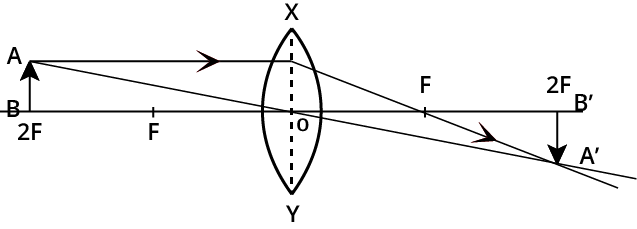Image source: Self-created

It is known that,

Magnification of a convex lens,$m=\frac{v}{u}$

$\Rightarrow -1=\frac{v}{u}$

$\Rightarrow -1=\frac{50}{u}$

$\Rightarrow u=-50cm$

Lens formula:$\frac{1}{v}-\frac{1}{u}=\frac{1}{f}$

$f$ is the focal length of the lens.

$\Rightarrow \frac{1}{f}=\frac{1}{50}-\frac{1}{(-50)}$

$\Rightarrow \frac{1}{f}=\frac{1}{50}+\frac{1}{50}$

$\Rightarrow \frac{1}{f}=\frac{2}{50}$

$\Rightarrow f=\frac{50}{2}$

$\Rightarrow f=25cm=0.25m$

It is known that,

Power of the lens,$P=\frac{1}{f(metres)}$

$\Rightarrow P=\frac{1}{(+0.25)}$

$\Rightarrow P=+4D$

Therefore, the object distance from the lens is $u=-50cm$ and power of the lens is $P=+4D$.

14. What is the power of a concave lens of focal length$2m$?

Ans: It is given that,

Focal length of a concave lens is,$f=-2m$

Power of the concave lens,$P=\frac{1}{f(metres)}$

$\Rightarrow P=\frac{1}{(-2)}$

$\Rightarrow P=-0.5D$

Therefore, the power of a concave lens is $P=-0.5D$.

15. Which one of the following materials cannot be used to make a lens?

1. Water

2. Glass

3. Plastic

4. Clay

Ans: d) Clay can’t be used to make a lens because it is opaque.

16. The image formed by a concave mirror is observed to be virtual, erect and larger than the object. Where should the position of the object be?

1. Between the principal focus and the centre of curvature

2. At the centre of curvature

3. Beyond the centre of curvature

4. Between the pole of the mirror and its principal focus.

Ans: d) The object is placed between the pole of the mirror and its principal focus when the image formed is virtual, erect and larger than the object in the concave mirror.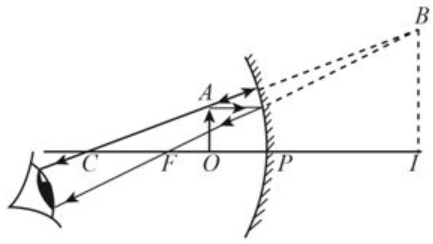17. Where should an object be placed in front of a convex lens to get a real image of the size of the object?

1. At the principal focus of the lens

2. At twice the focal length

3. At infinity

4. Between the optical centre of the lens and its principal focus

Ans: b) An object should be placed at a distance of twice the focal length of a convex lens to get a real image of the size of the object.18. A spherical mirror and a thin spherical lens have each a focal length of $-15cm$ . The mirror and the lens are likely to be

1. Both concave

2. Both convex

3. The mirror is concave and the lens is convex

4. The mirror is convex, but the lens is concave

Ans: a) For a concave lens the primary focus is on the same side as the object and is negative. In the case of a concave mirror, the focus is in front of the mirror and negative. Therefore, the mirror and lens are likely to be concave.

19. No matter how far you stand from a mirror, your image appears erect. The mirror is likely to be

1. Plane

2. Concave

3. Convex

4. Either plane or convex

Ans: d) Erect images are produced by both plane and convex mirrors for objects at any position.

20. Which of the following lenses would you prefer to use while reading small letters found in a dictionary?

1. A convex lens of focal length $\mathbf{50}\text{ }\mathbf{cm}$

2. A concave lens of focal length $\mathbf{50}\text{ }\mathbf{cm}$

3. A convex lens of focal length $\mathbf{5}\text{ }\mathbf{cm}$

4. A concave lens of focal length $\mathbf{5}\text{ }\mathbf{cm}$

Ans: a) When the object is placed between focus and optic centre, magnified and erect images are formed in a convex lens. So, while reading small letters a convex lens is preferred.

21. We wish to obtain an erect image of an object, using a concave mirror of focal length $\mathbf{15}\text{ }\mathbf{cm}$. What should be the range of distance of the object from the mirror? What is the nature of the image? Is the image larger or smaller than the object? Draw a ray diagram to show the image formation in this case.

Ans: To obtain an erect image in a concave mirror the object should be placed between Focus and the Optic centre.

Here, the focal length of the concave mirror is given as $15cm$.

Therefore, the range of distance of the object from the mirror is from $0cm$ to$15cm$.

The nature of the image is virtual.

The image is larger than the object.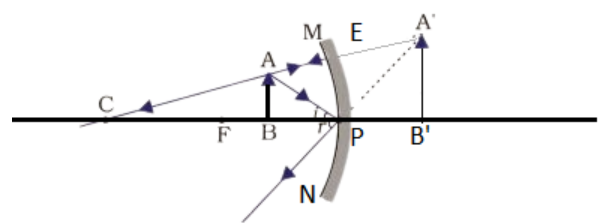A virtual, erect and magnified image is formed.

22. Name the type of mirror used in the following situations and support your answer with reason.

Ans: In the headlights of a car, a concave mirror is used. Because in concave mirrors a parallel beam of light is produced if the bulb is placed at the focus.

1. Side/Rear-View Mirror of a Vehicle

Ans: In a side/rear-view mirror of a vehicle , a convex mirror is used. Because when objects are placed in front of the convex mirror, erect and diminished images are formed which gives a wider field of view.

1. Solar Furnace

Ans: In solar furnaces, Concave mirrors are used. They converge sunlight to a point and produce high temperatures because of their converging properties.

23. One-half of a convex lens is covered with a black paper. Will this lens produce a complete image of the object? Verify your answer experimentally. Explain your observations.

Ans:  Yes, the lens produces a complete image of the object with less intensity.

Consider the following two cases: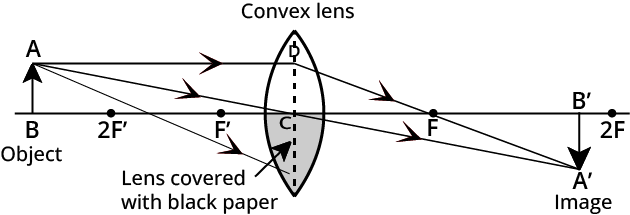In the first case the lower half of the lens is covered with black paper. Light rays coming from the object are refracted only from the upper half and the image is formed, whereas in the lower half the light rays are blocked.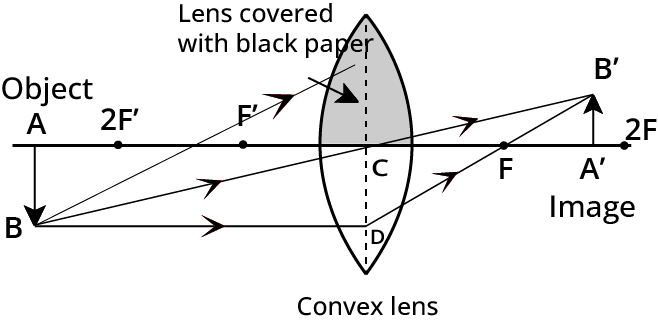In the second case the upper half of the lens is covered with black paper. Light rays coming from the object are refracted only from the lower half and the image is formed, whereas in the upper half the light rays are blocked.

Therefore, change in intensity of the image is observed i.e., the intensity of the image is less and the complete image is formed.

24. An object of $\mathbf{5}\text{ }\mathbf{cm}$ in length is held $\mathbf{25}\text{ }\mathbf{cm}$ away from a converging lens of focal length $\mathbf{10}\text{ }\mathbf{cm}$ . Draw the ray diagram and find the position, size and the nature of the image formed.

Ans: It is given that,

Height of the object,${{h}_{o}}=5cm$

Distance of object in front of lens,$u=-25cm$

Distance of image from lens,$v=?$

Focal length of the lens,$f=+10cm$

From lens formula:$\frac{1}{v}-\frac{1}{u}=\frac{1}{f}$

$\Rightarrow \frac{1}{v}=\frac{1}{f}+\frac{1}{u}$

$\Rightarrow \frac{1}{v}=\frac{1}{10}-\frac{1}{25}$

$\Rightarrow \frac{1}{v}=\frac{25-10}{250}$

$\Rightarrow \frac{1}{v}=\frac{15}{250}$

$\Rightarrow v=\frac{250}{15}$

$\Rightarrow v=16.66cm$

The positive value of $v$ indicates that the image and the object are on opposite sides.

It is known that,

Magnification ,$m=\frac{{{h}_{i}}}{{{h}_{o}}}=-\frac{v}{u}$

Height of the image, ${{h}_{i}}=?$

$\Rightarrow m=\frac{{{h}_{i}}}{5}=-\frac{16.66}{25}=-0.66$

$\Rightarrow {{h}_{i}}=-0.66\times 5$

$\Rightarrow {{h}_{i}}=-3.3cm$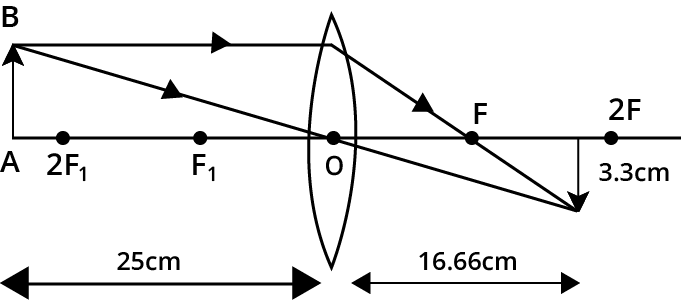As the magnification is $-0.66$, the negative sign indicates that the object is inverted and less than $1$ indicates that the image is smaller than the object.

Therefore, the position of the image is $16.66cm$ from the lens. The height of the object is $3.3cm$. The nature of the image is real, inverted and diminished.

25. A concave lens of focal length $\mathbf{15}\text{ }\mathbf{cm}$ forms an image $\mathbf{10}\text{ }\mathbf{cm}$ from the lens. Find the distance of an object from the lens? Draw the ray diagram.

Ans: It is given that,

Focal length of the lens,$f=-15cm$

Distance of image from lens,$v=-10cm$

Distance of object in front of lens,$u=?$

From lens formula:$\frac{1}{v}-\frac{1}{u}=\frac{1}{f}$

$\Rightarrow \frac{1}{u}=\frac{1}{v}-\frac{1}{f}$

$\Rightarrow \frac{1}{u}=\frac{1}{(-10)}-\frac{1}{(-15)}$

$\Rightarrow \frac{1}{u}=\frac{-1}{10}+\frac{1}{15}$

$\Rightarrow \frac{1}{u}=\frac{-5}{150}$

$\Rightarrow u=-\frac{150}{5}$

$\Rightarrow u=-30cm$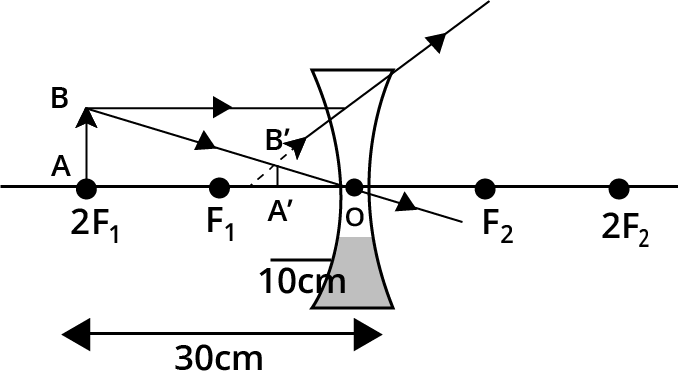Thus, the object is at a distance of $30cm$ from the lens.

26. An object is placed at a distance of $\mathbf{10}\text{ }\mathbf{cm}$ from a convex mirror of focal length $\mathbf{15}\text{ }\mathbf{cm}$ . Find the position and nature of the image.

Ans: It is given that,

Focal length of the convex mirror,$f=+15cm$

Distance of object in front of convex mirror,$u=-10cm$

Distance of image from convex mirror,$v=?$

From mirror formula:$\frac{1}{v}+\frac{1}{u}=\frac{1}{f}$

$\Rightarrow \frac{1}{v}=\frac{1}{f}-\frac{1}{u}$

$\Rightarrow \frac{1}{v}=\frac{1}{15}-\frac{1}{(-10)}$

$\Rightarrow \frac{1}{v}=\frac{1}{15}+\frac{1}{10}$

$\Rightarrow \frac{1}{v}=\frac{25}{150}$

$\Rightarrow v=\frac{150}{25}$

$\Rightarrow v=6cm$

The positive value of $v$ indicates that the image is formed behind the mirror and virtual.

It is known that,

Magnification ,$m=\frac{{{h}_{i}}}{{{h}_{o}}}=-\frac{v}{u}$

$\Rightarrow m=\frac{-6}{-10}=+0.6$

The positive magnification indicates that the image is erect. As magnification is less than $1$ it indicates that the image is smaller than the object.

Therefore, the position of image is $6cm$ behind the mirror. Nature of image is virtual, erect and diminished.

27. What does “The magnification produced by a plane mirror is $+1$ ” mean?

Ans: It is known that,

Magnification produced by a plane mirror is,$m=\frac{{{h}_{i}}}{{{h}_{o}}}=-\frac{v}{u}$

where,

${{h}_{i}}$ is the height of the image

${{h}_{o}}$ is the height of the object

$u$ is the distance of the object in front of lens

$v$ is the distance of image from lens

Magnification produced by a plane mirror is $+1$ means that ${{h}_{i}}={{h}_{o}}$ that is the size of the image is the same as the size of the object. The positive size indicates that the image is erect.

Therefore, magnification equal to $+1$ means that the size of the image is the same as object and erect.

28. An object $\mathbf{5}\text{ }\mathbf{cm}$ in length is placed at a distance of $\mathbf{20}\text{ }\mathbf{cm}$in front of a convex mirror of radius of curvature$\mathbf{30}\text{ }\mathbf{cm}$ . Find the position of the image, its nature and size.

Ans: It is given that,

Distance of object in front of the mirror,$u=-20cm$

Distance of image from the mirror,$v=?$

The radius of curvature of the mirror,$R=30cm$

The focal length of the mirror,$f=?$

It is known that,

Radius of curvature is equal to twice the focal length.

$\Rightarrow R=2f$

$\Rightarrow 30=2f$

$f=\frac{30}{2}=15cm$

From mirror formula:$\frac{1}{v}+\frac{1}{u}=\frac{1}{f}$

$\Rightarrow \frac{1}{v}=\frac{1}{f}-\frac{1}{u}$

$\Rightarrow \frac{1}{v}=\frac{1}{15}-\frac{1}{(-20)}$

$\Rightarrow \frac{1}{v}=\frac{1}{15}+\frac{1}{20}$

$\Rightarrow \frac{1}{v}=\frac{20+15}{300}$

$\Rightarrow \frac{1}{v}=\frac{35}{300}$

$\Rightarrow v=\frac{60}{7}$

$\Rightarrow v=8.57cm$

The positive value of $v$ indicates that the image is formed behind the mirror.

It is known that,

Magnification ,$m=\frac{{{h}_{i}}}{{{h}_{o}}}=-\frac{v}{u}$

Height of the object, ${{h}_{o}}=5cm$

Height of the image, ${{h}_{i}}=?$

$\Rightarrow {{h}_{i}}=-\frac{v}{u}\times {{h}_{o}}$

$\Rightarrow {{h}_{i}}=-\frac{8.57}{(-20)}\times 5$

$\Rightarrow {{h}_{i}}=2.14cm$

Therefore, the image is formed at a distance of $8.57cm$behind the mirror. The nature of the image is virtual, erect and diminished.

29. An object of size $\mathbf{7}.\mathbf{0}\text{ }\mathbf{cm}$ is placed at $\mathbf{27}\text{ }\mathbf{cm}$ in front of a concave mirror of focal length $\mathbf{18}\text{ }\mathbf{cm}$ . At what distance from the mirror should a screen be placed, so that a sharp focused image can be obtained? Find the size and the nature of the image.

Ans: It is given that,

Distance of object in front of mirror,$u=-27cm$

Distance of image from the mirror,$v=?$

Focal length of the mirror,$f=-18cm$

From mirror formula:$\frac{1}{v}+\frac{1}{u}=\frac{1}{f}$

$\Rightarrow \frac{1}{v}=\frac{1}{f}-\frac{1}{u}$

$\Rightarrow \frac{1}{v}=\frac{1}{-18}-\frac{1}{(-27)}$

$\Rightarrow \frac{1}{v}=-\frac{1}{18}+\frac{1}{27}$

$\Rightarrow \frac{1}{v}=\frac{-3+2}{54}$

$\Rightarrow \frac{1}{v}=-\frac{1}{54}$

$\Rightarrow v=-54cm$

The negative value of $v$ indicates that the screen should be placed at a distance of  $54cm$ in front of the mirror and the image is real.

It is known that,

Magnification ,$m=\frac{{{h}_{i}}}{{{h}_{o}}}=-\frac{v}{u}$

Height of the object, ${{h}_{o}}=7cm$

Height of the image, ${{h}_{i}}=?$

$\Rightarrow {{h}_{i}}=-\frac{v}{u}\times {{h}_{o}}$

$\Rightarrow {{h}_{i}}=-\frac{-54}{(-27)}\times 7$

$\Rightarrow {{h}_{i}}=-14cm$

The height of image is $14cm$.

Therefore, the image is formed at a distance of $54cm$in front of the mirror. Nature of image is real, inverted and enlarged.

30. Find the focal length of a lens of power$-\mathbf{2}.\mathbf{0}\text{ }\mathbf{D}$ . What type of lens is this?

Ans: It is given that,

Power of a lens,$P=-2.0D$

Focal length of a lens, $f=?$

Power of a lens,$P=\frac{1}{f(metres)}$

$\Rightarrow -2=\frac{1}{f}$

$\Rightarrow f=-\frac{1}{2}=-0.5m$

Negative $f$ indicates concave lens.

Therefore, the focal length of lens is $f=-0.5m$and the lens is concave.

31. A doctor has prescribed a corrective lens of power $+\mathbf{1}.\mathbf{5}\text{ }\mathbf{D}$. Find the focal length of the lens. Is the prescribed lens diverging or converging?

Ans: It is given that,

Power of a lens,$P=+1.5D$

Focal length of a lens, $f=?$

Power of a lens,$P=\frac{1}{f(metres)}$

$\Rightarrow 1.5=\frac{1}{f}$

$\Rightarrow f=\frac{1}{1.5}=0.66m$

Positive $f$ indicates a convex lens.

Therefore, the focal length of lens is $f=0.66m$ and the lens prescribed is a diverging lens.

### NCERT Solutions for Class 10 Science Chapter 10 Light Reflection and Refraction - Free PDF Download

It is quite necessary to have at least basic knowledge of Science which may help you in the future. Class 10 Science Chapter 10 NCERT Solutions are structured in a comprehensive and presentable way to provide students with complete coverage of the topics and scientific terms which can help students in scoring well in their exams as well as gain perspective towards career goals. Opting for Light Reflection and Refraction Class 10 NCERT Solutions can help a student in ensuring excellent performance in your exams. This free PDF contains solutions to all problems in Chapter 10 Class 10 Science.

### NCERT Solutions For Science Class 10 - Light Reflection and Refraction

Chapter 10 Class 10 Science is mostly about reflection and refraction of light. The main topics included consist of spherical mirrors, magnification, reflection, refraction and power of a lens. To provide simplification, Light Reflection and Refraction Class 10 Solutions include various diagrams, logical problems and detailed content in simple words.

The topic Reflection of Light Class 10 refers to light waves bouncing off the surface of objects that do not have the capability of absorbing energy produced by radiation. A surface of a pool, for example, will display clarity of surrounding objects when still. Whereas, all the rays would be scattered once a rock is thrown inside it preventing systematic reflection of light.

Refraction of light Class 10, on the other hand, refers to the bending of light waves when it travels from a faster to a slower medium. Images formed by lenses and our eyes exist due to this process.

All the aforementioned topics are thoroughly explained under Chapter 10 Science Class 10 solutions by teachers having expertise in the subject. Physics subject requires students to have a good grip on the basic understanding of the concepts. NCERT Solutions for Class 10 Science Chapter 10 provides an easy understanding of the various topics that can help students to secure good marks.

### Class 10 Science Chapter 10 Weightage Marks

Light Reflection and Refraction Class 10 is one of the significant sections in Class 10 Science. The normal weightage of the part as indicated by the most recent guidelines is 7 marks. In Ch 10 Science Class 10, students will get knowledge about refraction and reflection. One will find out about spherical mirrors, the formation of images and draw ray diagrams.

Following are the topics covered under Chapter 10 Class 10 Science:

Ex 10.1 - Reflection of Light.

Ex 10.2 - Spherical Mirrors.

Ex 10.3 - Image Formation by Spherical Mirrors.

Ex 10.4 - Representation of Images formed by Spherical Mirrors using Ray diagrams (2 long answer and 2 short answer questions).

Ex 10.5 - Mirror Formula and Magnification (2 numerical questions).

Ex 10.6 - Refraction of Light (1 short and 2 long answers, 1 numerical).

Ex 10.7 - Power of Lens (1 numerical and 2 short answers).

### Benefits of Light Reflection and Refraction Class 10 solutions

Class 10th Science Chapter 10 offers great opportunities for students towards achieving maximum marks. To do so, students must have a good understanding of the concepts of this chapter which is duly sufficed by NCERT Solutions for Class 10 Science Chapter 10. The main benefits of these solutions are:

• Chapter 10 Science Class 10 NCERT Solutions are given by faculties who are specialists in this field and have an excellent level of experience. The solutions created by them are exact and will help you in making sure that you secure good marks in the tests.

• The solutions to various questions in this chapter are clarified with charts and viable diagrams with regards to an easy understanding of students.

• The solutions are designed and created keeping in mind one of the main objectives of helping students in obtaining high marks.

• The solutions are not very complicated and explain step-by-step processes thoroughly to help students have a strong understanding of the concepts. The language utilized is clear and can be perceived by all.

• The solutions provided are most likely to come for tests and exams.

### Key Features of NCERT Solutions Class 10 Science Chapter 10

Have you ever wondered what allows us to see things? The answer is Light. During the day, sunlight helps us see things better. When the light touches an item, it reflects. This reflected light helps us to see the objects when it reaches our eyes. Many phenomena are caused by light, including the formation of the rainbow, stars. In Class 10 Science Chapter 10 Light Reflection and Refraction, we'll study the processes of refraction and reflection using the straight-line light transmission. The key features of the NCERT Solutions Class 10 Science Chapter 10 are as follows.

• Provides thorough answers to all questions of the chapter.

• The information provided is authentic, and the language used is clear and understandable to all.

• For Olympiads, CBSE exams, and other competitive exams, these solutions can be used.

### Practice Questions for Class 10 Science Maths Chapter 10

1. What is the principal focus of a concave mirror?

2. What are spherical mirrors?

3. How is the image formed by concave mirrors and convex mirrors? Explain with diagrams.

4. What would be the focal length of a concave mirror whose radius of curvature is 32 cm?

5. What is the lens formula?

6. Why are convex mirrors preferred as rear-view mirrors in vehicles?

7. What is the double convex lens?

8. What is the speed of light in rarer medium and denser medium?

9. Is there any relationship between the radius of curvature  and the focal length (F) of a spherical mirror?

10. Define one diopter of the power of the lens.

NCERT Solutions for Class 10 Science Chapter 10 will be beneficial for the students of Class 10 CBSE. The students are required to use these NCERT Solutions after understanding all the topics of the chapter thoroughly to brush their understanding and at the time of their revision. They must rely on the free PDF as the NCERT solutions are authentic and are curated by the best teachers after thorough research.

Also Access

## FAQs on NCERT Solutions for Class 10 Science Chapter 10 - Light Reflection And Refraction

1. Why do Vehicles have Convex-Shaped Rear View Mirrors?

A convex mirror is used as a rearview mirror in vehicles as it gives a more extensive field of view, which causes the driver to see the vast majority of the traffic behind him. Convex mirrors consistently structure an erect, virtual and decreased picture of the things set in front of it.

2. What is Meant by 1 Dioptre of Power of Lens?

Dioptre is the SI unit of intensity of focal point and is denoted by the letter D. 1 dioptre can be characterized as the intensity of a focal point/power of a lens of central length 1 metre.

3. What are the concepts covered in Chapter 10 of Class 10 Science?

The concepts included in this chapter are:

Reflection of Light

• Spherical Mirrors

• Image Formation by Spherical Mirrors

• Representation of Images Formed by Spherical Mirrors Using Ray Diagrams

• Sign Convention for Reflection by Spherical Mirrors

• Mirror Formula and Magnification

Refraction of Light

• Refraction through a Rectangular Glass Slab

• The Refractive Index

• Refraction by Spherical Mirrors

• Image Formation by Lenses

• Image Formation by Lenses Using Ray Diagrams

• Sign Convention for Spherical Lenses

• Lens Formula and Magnification

• Power of a Lens

4. Where can I get the NCERT Solutions of Chapter 10 of Class 10 Science?

Students will find the NCERT Solutions of Chapter 10 of Class 10 Science on the website of Vedantu.

• Visit the page-NCERT Solutions for Chapter 10 of Class 10 Science.

• This link will take you to the official webpage of Vedantu (vedantu.com).

• There you will find the PDF file of the NCERT Solution of Chapter 10 of Class 10 Science.

• Click on the option of “Download PDF” which is at the top of the PDF file.

• The file will get downloaded free of cost after clicking the option.

5. What do you understand about the refraction of light? Also, state the laws of refraction of light?

Refraction of light is the process in which the direction and speeds of light change when moving from one medium to another.

Laws of Refraction of Light:

• The normal, the incident ray and the refracted ray all lie in the same plane.

• The ratio of the sine of the angle of incidence to the sine of the angle of refraction is a constant for the light of a given colour and for the given pair of media. This law is known as Snell's law.

6. What is meant by the term magnification of spherical mirrors?

The magnification of spherical mirrors is defined as the ratio of the height of the image to the height of the object. It is denoted by ‘m’. This magnification is also described in terms of object and image distance. The negative ratio of the image distance to the object distance is known as magnification. The negative magnification shows that images are real. The positive sign in the value of magnification tells that the image is virtual.

7. Write a short note on the power of a lens?

The power of a lens is defined as the ability of the lens to diverge or converge the light rays depending on the focal length. The short focal length of the concave lens causes higher divergence than the one with a longer focal length. The power of the lens is also defined as the reciprocal of its focal length. It is denoted by “P”. The SI unit of power of the lens is ‘dioptre’. 1 dioptre is described as the power of a lens whose focal length is 1 metre.